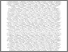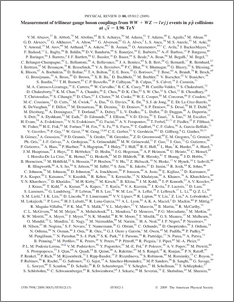# Measurement of trilinear gauge boson couplings from WW + WZ to lnu jj events in pp-bar collisions at sqrt{s}=1.96 TeV

D0 Collaboration, The and Bertram, Iain and Borissov, Guennadi and Fox, Harald and Williams, Mark and Ratoff, Peter and Love, Peter and Rakitin, Alexander and Sopczak, Andre (2009) Measurement of trilinear gauge boson couplings from WW + WZ to lnu jj events in pp-bar collisions at sqrt{s}=1.96 TeV. Physical Review D, 80 (5). ISSN 1550-7998Preview
PDF
PhysRevD.80.053012.pdf - Published Version

## Abstract

We present a direct measurement of trilinear gauge boson couplings at gammaWW and ZWW vertices in WW and WZ events produced in pp-bar collisions at sqrt{s}=1.96 TeV. We consider events with one electron or muon, missing transverse energy, and at least two jets. The data were collected using the D0 detector and correspond to 1.1/fb of integrated luminosity. Considering two different relations between the couplings at the gammaWW and ZWW vertices, we measure these couplings at 68% C.L. to be kappa_{gamma}=1.07^{+0.26}_{-0.29}, lambda =0.00^{+0.06}_{-0.06} and g_{1}^{Z}=1.04^{+0.09}_{-0.09} in a scenario respecting SU(2)_L x U(1)_Y gauge symmetry and kappa =1.04^{+0.11}_{-0.11} and lambda=0.00^{+0.06}_{-0.06} in an "equal couplings" scenario.

Item Type:
Journal Article
Journal or Publication Title:
Physical Review D